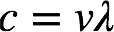# Problem: Photosynthesis uses 660-nm light to convert CO 2 and H2O into glucose and O 2. Calculate the frequency of this light.

###### FREE Expert Solution

Recall: The relationship between frequency ν and wavelength λ is:where c = speed of light, 3.00 × 108 m • s–1. We're given light with a wavelength = 660 nm.

98% (312 ratings)###### Problem Details

Photosynthesis uses 660-nm light to convert CO 2 and H2O into glucose and O 2. Calculate the frequency of this light.